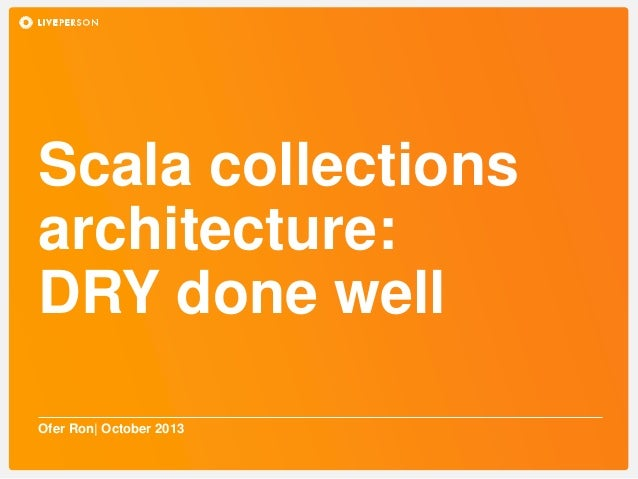Successfully reported this slideshow.Upcoming SlideShare
×

Scala Collections Architecture: DRY Done Well

937 views

Published on

Hello all,
My name is Ofer Ron, and I'm a senior data scientist at LivePerson.

Scala's collections library is a very good example of the programming maxim of Don't Repeat Yourself, or DRY. Using Scala's strong type system and orthogonal design principles, it enables code reuse between different collection types, while keeping the API frictionless.
In my talk, which follows the paper "Fighting Bit Rot with Types" (http://lampwww.epfl.ch/~odersky/papers/fsttcs2009.pdf) by the library designers, I demonstrated the principles behind the design, and the type system tricks which enable them.
Video: http://www.parleys.com/play/5281ffc0e4b054cd7d2ef319

Published in: Technology
• Full Name
Comment goes here.

Are you sure you want to Yes No• Be the first to comment

Scala Collections Architecture: DRY Done Well

1. 1. Scala collections architecture: DRY done well Ofer Ron| October 2013
2. 2. Huh? Our goal is understanding: def map[B, That](f: A => B) (implicit bf: CanBuildFrom[Repr, B, That]): That
3. 3. The collection traits
4. 4. Traversable – the wanted behavior trait Traversable[+A] { def foreach[U](f:A=>U) def filter(p:A=>Boolean):??? } What’s the right return type?
5. 5. The challenge The reasonable behavior List[A] List[A] filter(f:A=>Boolean) Bonus question: Set[A] Set[A] filter(f:A=>Boolean) String String filter(f:Char=>Boolean)
6. 6. Building every type class Builder[-Elem,+To] { def +=(elem: Elem): this.type = . . . def result(): To = . . . def clear() = . . . }
7. 7. TraversableLike – abstractions trait TraversableLike[+Elem,+Repr] { def newBuilder:Builder[Elem,Repr] def foreach[U](f:Elem=>U) def filter(p:Elem=>Boolean):Repr = { val b = newBuilder foreach (e => if (p(e)) b+= e) b.result } }
8. 8. TraversableLike – abstractions trait Traversable[+Elem] extends TraversableLike[Elem,Traversable[Elem]] {…} Abstract on implementation and type
9. 9. We can filter, now what? The reasonable behavior for map List[A] List[B] map(f:A=>B) Set[A] Set[B] map(f:A=>B)
10. 10. Can the old solution work? BitSet BitSet Set[String]
11. 11. Can the old solution work? – another example Map[A,B] Map[C,D] Iterable[C]
12. 12. Aside - Functional dependencies trait Matrix, trait Vector Matrix * Vector => Vector Matrix * Matrix => Matrix Int * Matrix => Matrix Vector * Vector => undefined
13. 13. Aside - Functional dependencies – cnt’d trait Mult [A,B,C] { def apply(a:A,b:B):C } def multiply[A,B,C](a:A,b:B)(implicit mult:Mult[A,B,C]) = mult(a,b) implicit object MatrixVectorMult extends Mult[Matrix,Vector,Vector]
14. 14. CanBuildFrom - Using Functional dependencies trait TraversableLike[+A,+Repr] { def map[B, That](f: A => B) (implicit bf: CanBuildFrom[Repr, B, That]): That = ??? } trait CanBuildFrom[-Collection, -NewElem, +Result] { def apply(from: Collection): Builder[NewElem, Result] }
15. 15. The BitSet hierarchy TraversableLike[+A,+Repr] SetLike[A,+Repr] BitSetLike[+This <: BitSetLike[This] with Set[Int]] Traversable[+A] Set[A] BitSet A=Int A=Int
16. 16. CanBuildFrom - Using Functional dependencies object Set { implicit def canBuildFromSet[B] = new CanBuildFrom[Set[_], B, Set[B]] {…} } object BitSet { implicit def canBuildFromBitSet[B] = new CanBuildFrom[BitSet, Int, BitSet] {…} }
17. 17. Static vs dynamic type val els:Iterable[Int] = List(1,2,3) Static type Dynamic type def map[A,B](els:Iterable[A])(f:A=>B):Iterable[B] = els map f val newEls:Iterable[String] = map(List(1,2,3))(_.toString) We want dynamic type list
18. 18. Static vs dynamic type – cnt’d Compiler will capture: CanBuildFrom[Iterable[_],B,Iterable[B]] We can follow the call chain to understand how the correct dynamic type can be created
19. 19. Static vs dynamic type – cnt’d New Coursera course: Principles of Reactive Programming Martin Odersky, Erik Meijer and Roland Kuhn Nov. 4th, 2013
20. 20. Thank You We’re hiring!